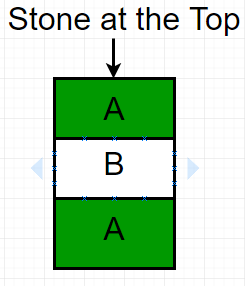# Game of Nim with removal of one stone allowed

• Difficulty Level : Basic
• Last Updated : 14 Apr, 2021

In Game of Nim, two players take turns removing objects from heaps or the pile of stones.
Suppose two players A and B are playing the game. Each is allowed to take only one stone from the pile. The player who picks the last stone of the pile will win the game. Given N the number of stones in the pile, the task is to find the winner, if player A starts the game.
Examples :

```Input : N = 3.
Output : Player A``````Player A remove stone 1 which is at the top, then Player B remove stone 2
and finally player A removes the last stone.

Input : N = 15.
Output : Player A```

## Recommended: Please try your approach on {IDE} first, before moving on to the solution.

For N = 1, player A will remove the only stone from the pile and wins the game.
For N = 2, player A will remove the first stone and then player B remove the second or the last stone. So player B will win the game.
So, we can observe player A wins when N is odd and player B wins when N is even.
Below is the implementation of this approach:

## C++

 `// C++ program for Game of Nim with removal``// of one stone allowed.``#include``using` `namespace` `std;` `// Return true if player A wins,``// return false if player B wins.``bool` `findWinner(``int` `N)``{``  ``// Checking the last bit of N.``  ``return` `N&1;``}` `// Driven Program``int` `main()``{``  ``int` `N = 15;``  ``findWinner(N)? (cout << ``"Player A"``;):``                 ``(cout << ``"Player B"``;);``  ``return` `0;``}`

## Java

 `// JAVA Code For Game of Nim with``// removal of one stone allowed``import` `java.util.*;` `class` `GFG {``    ` `    ``// Return true if player A wins,``    ``// return false if player B wins.``    ``static` `int` `findWinner(``int` `N)``    ``{``      ``// Checking the last bit of N.``      ``return` `N & ``1``;``    ``}``    ` `    ``/* Driver program to test above function */``    ``public` `static` `void` `main(String[] args)``    ``{``        ``int` `N = ``15``;``        ``if``(findWinner(N)==``1``)``            ``System.out.println(``"Player A"``);``        ``else``             ``System.out.println(``"Player B"``);``          ` `    ``}``}` `// This code is contributed by Arnav Kr. Mandal.`

## Python3

 `# Python3 code for Game of Nim with``# removal of one stone allowed.` `# Return true if player A wins,``# return false if player B wins.``def` `findWinner( N ):``    ` `    ``# Checking the last bit of N.``    ``return` `N & ``1``    ` `# Driven Program``N ``=` `15``print``(``"Player A"` `if` `findWinner(N) ``else` `"Player B"``)` `# This code is contributed by "Sharad_Bhardwaj".`

## C#

 `// C# Code For Game of Nim with``// removal of one stone allowed``using` `System;` `class` `GFG {``    ` `    ``// Return true if player A wins,``    ``// return false if player B wins.``    ``static` `int` `findWinner(``int` `N)``    ``{``        ``// Checking the last bit of N.``        ``return` `N & 1;``    ``}``    ` `    ``/* Driver program to test above function */``    ``public` `static` `void` `Main()``    ``{``        ``int` `N = 15;``        ` `        ``if``(findWinner(N) == 1)``            ``Console.Write(``"Player A"``);``        ``else``            ``Console.Write(``"Player B"``);``        ` `    ``}``}` `// This code is contributed by vt_m.`

## PHP

 ``

## Javascript

 ``

Output :

`Player A`

Time Complexity: O(1).
This article is contributed by Anuj Chauhan. If you like GeeksforGeeks and would like to contribute, you can also write an article using write.geeksforgeeks.org or mail your article to review-team@geeksforgeeks.org. See your article appearing on the GeeksforGeeks main page and help other Geeks.